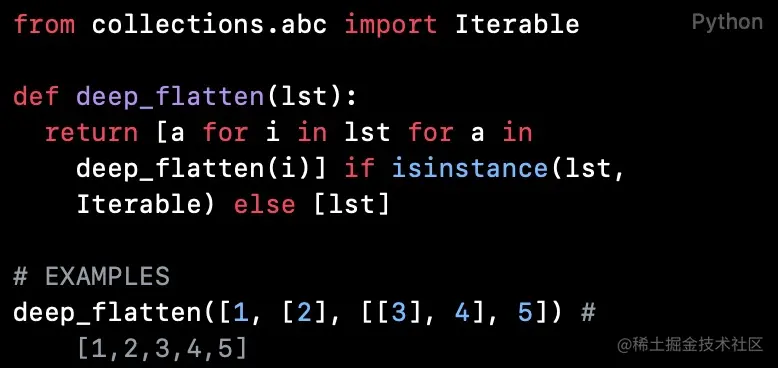# Python code reading (chapter 35): fully (deeply) expand nested lists

2022-01-29 11:08:15 FelixZPython Code reading collection Introduction ： Why not recommend Python Beginners directly look at the project source code

The code read in this article realizes the complete expansion of all nesting levels of a nested list , The function of forming a simple list .

## `deep_flatten`

``````from collections.abc import Iterable

def deep_flatten(lst):
return [a for i in lst for a in deep_flatten(i)] if isinstance(lst, Iterable) else [lst]

# EXAMPLES
deep_flatten([1, , [, 4], 5]) # [1,2,3,4,5]
Copy code ``````

`deep_flatten` The function receives a nested list , Return to the fully expanded list . Function USES `isinstance()` And `collections.abc.Iterable` To check whether an element is iteratable （ Is it `list`）. If it is , Recursively call... In the list derivation `deep_flatten()` function , Otherwise return to `[lst]`.

Primitive function ：

``````def deep_flatten(lst):
return [a for i in lst for a in deep_flatten(i)] if isinstance(lst, Iterable) else [lst]
Copy code ``````

It can be rewritten as ：

``````def deep_flatten(lst):
if isinstance(lst, Iterable):
return [a for i in lst for a in deep_flatten(i)]
else:
return [lst]
Copy code ``````

Function judgment if `lst` If it's an iteratable object , Is executed `return [a for i in lst for a in deep_flatten(i)]`. At this moment if `i` Is an iterable object , stay `deep_flatten(i)` The list derivation will continue to be called in , Continue to expand the nested list ; If `i` It's not an iterative object , In `deep_flatten(i)` And you'll come back `[i]`, here `a` The value is `i`, In the list derivation, you will get the element of a non iteratable object , Untie the nesting layer on the element .

A step closer , The function can be rewritten as ：

``````from collections.abc import Iterable

def deep_flatten(lst):
temp = []
def f(lst):
if isinstance(lst, Iterable):
for i in lst:
for a in f(i):
temp.append(a)
return []
else:
return [lst]
f(lst)
return temp

print(deep_flatten([1, , [, 4], 5])) # [1,2,3,4,5]
Copy code ``````